# 從零開始PyTorch項目：YOLO v3目標檢測實現

2018-04-23

https://github.com/ayooshkathuria/YOLO_v3_tutorial_from_scratch

1. YOLO 的工作原理

2. 創建 YOLO 網絡層級

3. 實現網絡的前向傳播

4. objectness 置信度閾值和非極大值抑制

5. 設計輸入和輸出管道

• 卷積神經網絡的工作原理，包括殘差塊、跳過連接和上採樣；

• 目標檢測、邊界框迴歸、IoU 和非極大值抑制；

• 基礎的 PyTorch 使用。你需要能夠輕鬆地創建簡單的神經網絡。

## 什麼是 YOLO？

YOLO 是 You Only Look Once 的縮寫。它是一種使用深度卷積神經網絡學得的特徵來檢測對象的目標檢測器。在我們上手寫代碼之前，我們必須先了解 YOLO 的工作原理。

YOLO 僅使用卷積層，這就使其成爲全卷積神經網絡（FCN）。它擁有 75 個卷積層，還有跳過連接和上採樣層。它不使用任何形式的池化，使用步幅爲 2 的卷積層對特徵圖進行下采樣。這有助於防止通常由池化導致的低級特徵丟失。

YOLO 通過被步幅對圖像進行上採樣。例如，如果網絡的步幅是 32，則大小爲 416×416 的輸入圖像將產生 13×13 的輸出。通常，網絡層中的任意步幅都指層的輸入除以輸入。

1×1×（B×（5+C））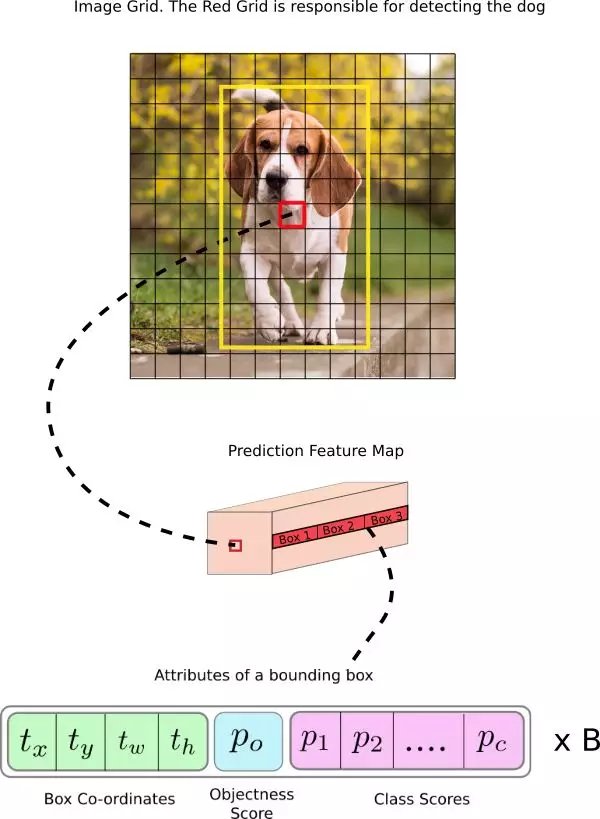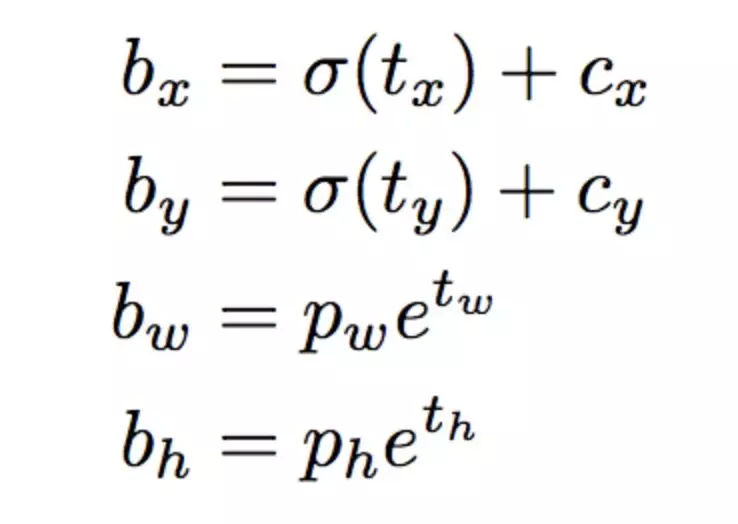• 與預測目標的網格單元左上角相關的偏移；

• 使用特徵圖單元的維度（1）進行歸一化的偏移。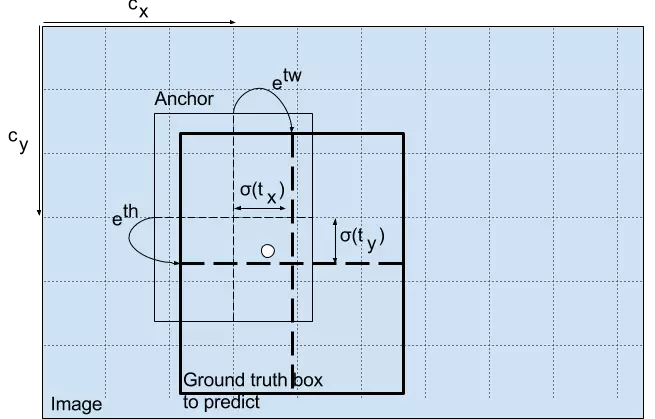Objectness 分數

Object 分數表示目標在邊界框內的概率。紅色網格和相鄰網格的 Object 分數應該接近 1，而角落處的網格的 Object 分數可能接近 0。

objectness 分數的計算也使用 sigmoid 函數，因此它可以被理解爲概率。

YOLO v3 在 3 個不同尺度上進行預測。檢測層用於在三個不同大小的特徵圖上執行預測，特徵圖步幅分別是 32、16、8。這意味着，當輸入圖像大小是 416 x 416 時，我們在尺度 13 x 13、26 x 26 和 52 x 52 上執行檢測。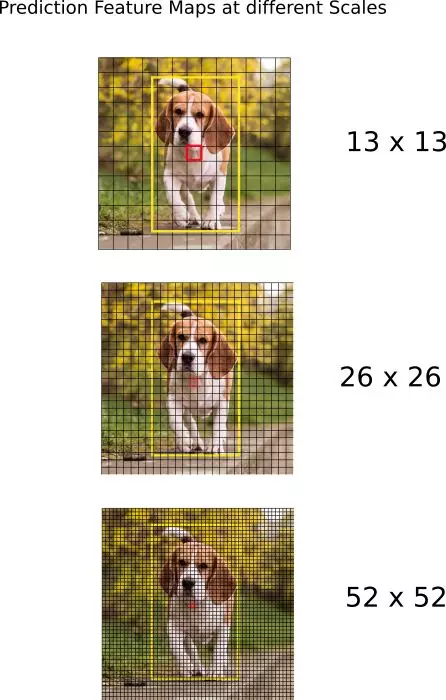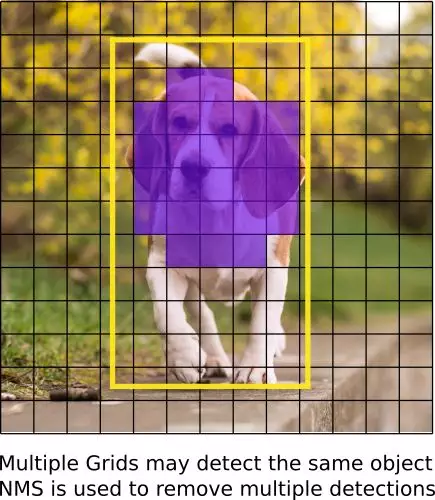YOLO 只能檢測出屬於訓練所用數據集中類別的對象。我們的檢測器將使用官方權重文件，這些權重通過在 COCO 數據集上訓練網絡而獲得，因此我們可以檢測 80 個對象類別。

1. YOLO V1: You Only Look Once: Unified, Real-Time Object Detection (https://arxiv.org/pdf/1506.02640.pdf)

2. YOLO V2: YOLO9000: Better, Faster, Stronger (https://arxiv.org/pdf/1612.08242.pdf)

3. YOLO V3: An Incremental Improvement (https://pjreddie.com/media/files/papers/YOLOv3.pdf)

4. Convolutional Neural Networks (http://cs231n.github.io/convolutional-networks/)

5. Bounding Box Regression (Appendix C) (https://arxiv.org/pdf/1311.2524.pdf)

6. IoU (https://www.youtube.com/watch?v=DNEm4fJ-rto)

7. Non maximum suppresion (https://www.youtube.com/watch?v=A46HZGR5fMw)

8. PyTorch Official Tutorial (http://pytorch.org/tutorials/beginner/deep_learning_60min_blitz.html)

## 第二部分：創建 YOLO 網絡層級

``mkdir cfgcd cfgwget https://raw.githubusercontent.com/pjreddie/darknet/master/cfg/yolov3.cfg``

``[convolutional]batch_normalize=1filters=64size=3stride=2pad=1activation=leaky[convolutional]batch_normalize=1filters=32size=1stride=1pad=1activation=leaky[convolutional]batch_normalize=1filters=64size=3stride=1pad=1activation=leaky[shortcut]from=-3activation=linear``

1. 卷積層

``[convolutional]batch_normalize=1 filters=64 size=3 stride=1 pad=1 activation=leaky``

2. 跳過連接

``[shortcut]from=-3 activation=linear ``

3.上採樣

``[upsample]stride=2``

4.路由層（Route）

``[route]layers = -4[route]layers = -1, 61``

5.YOLO

``[yolo]mask = 0,1,2anchors = 10,13, 16,30, 33,23, 30,61, 62,45, 59,119, 116,90, 156,198, 373,326classes=80num=9jitter=.3ignore_thresh = .5truth_thresh = 1random=1``

YOLO 層級對應於上文所描述的檢測層級。參數 anchors 定義了 9 組錨點，但是它們只是由 mask 標籤使用的屬性所索引的錨點。這裏，mask 的值爲 0、1、2 表示了第一個、第二個和第三個使用的錨點。而掩碼錶示檢測層中的每一個單元預測三個框。總而言之，我們檢測層的規模爲 3，並裝配總共 9 個錨點。

Net

``[net]# Testingbatch=1subdivisions=1# Training# batch=64# subdivisions=16width= 320height = 320channels=3momentum=0.9decay=0.0005angle=0saturation = 1.5exposure = 1.5hue=.1``

``from __future__ import divisionimport torch import torch.nn as nnimport torch.nn.functional as F from torch.autograd import Variableimport numpy as np``

``def parse_cfg(cfgfile): """ Takes a configuration file Returns a list of blocks. Each blocks describes a block in the neural network to be built. Block is represented as a dictionary in the list """``

``file = open(cfgfile, 'r')lines = file.read().split('\n') # store the lines in a listlines = [x for x in lines if len(x) > 0] # get read of the empty lines lines = [x for x in lines if x != '#'] # get rid of commentslines = [x.rstrip().lstrip() for x in lines] # get rid of fringe whitespaces``

``block = {}blocks = []for line in lines: if line == "[": # This marks the start of a new block if len(block) != 0: # If block is not empty, implies it is storing values of previous block. blocks.append(block) # add it the blocks list block = {} # re-init the block block["type"] = line[1:-1].rstrip()  else: key,value = line.split("=")  block[key.rstrip()] = value.lstrip()blocks.append(block)return blocks``

create_modules 函數使用 parse_cfg 函數返回的 blocks 列表：

``def create_modules(blocks): net_info = blocks #Captures the information about the input and pre-processing  module_list = nn.ModuleList() prev_filters = 3 output_filters = []``

nn.ModuleList

`` for index, x in enumerate(blocks[1:]): module = nn.Sequential() #check the type of block #create a new module for the block #append to module_list``

nn.Sequential 類被用於按順序地執行 nn.Module 對象的一個數字。如果你查看 cfg 文件，你會發現，一個模塊可能包含多於一個層。例如，一個 convolutional 類型的模塊有一個批量歸一化層、一個 leaky ReLU 激活層以及一個卷積層。我們使用 nn.Sequential 將這些層串聯起來，得到 add_module 函數。例如，以下展示了我們如何創建卷積層和上採樣層的例子：

`` if (x["type"] == "convolutional"): #Get the info about the layer activation = x["activation"] try: batch_normalize = int(x["batch_normalize"]) bias = False except: batch_normalize = 0 bias = True filters= int(x["filters"]) padding = int(x["pad"]) kernel_size = int(x["size"]) stride = int(x["stride"]) if padding: pad = (kernel_size - 1) // 2 else: pad = 0 #Add the convolutional layer conv = nn.Conv2d(prev_filters, filters, kernel_size, stride, pad, bias = bias) module.add_module("conv_{0}".format(index), conv) #Add the Batch Norm Layer if batch_normalize: bn = nn.BatchNorm2d(filters) module.add_module("batch_norm_{0}".format(index), bn) #Check the activation.  #It is either Linear or a Leaky ReLU for YOLO if activation == "leaky": activn = nn.LeakyReLU(0.1, inplace = True) module.add_module("leaky_{0}".format(index), activn) #If it's an upsampling layer #We use Bilinear2dUpsampling elif (x["type"] == "upsample"): stride = int(x["stride"]) upsample = nn.Upsample(scale_factor = 2, mode = "bilinear") module.add_module("upsample_{}".format(index), upsample)``

`` #If it is a route layer elif (x["type"] == "route"): x["layers"] = x["layers"].split(',') #Start of a route start = int(x["layers"]) #end, if there exists one. try: end = int(x["layers"]) except: end = 0 #Positive anotation if start > 0:  start = start - index if end > 0: end = end - index route = EmptyLayer() module.add_module("route_{0}".format(index), route) if end < 0: filters = output_filters[index + start] + output_filters[index + end] else: filters= output_filters[index + start] #shortcut corresponds to skip connection elif x["type"] == "shortcut": shortcut = EmptyLayer() module.add_module("shortcut_{}".format(index), shortcut)``

``route = EmptyLayer()``

``class EmptyLayer(nn.Module): def __init__(self): super(EmptyLayer, self).__init__()``

``if end < 0: #If we are concatenating maps filters = output_filters[index + start] + output_filters[index + end]else: filters= output_filters[index + start]``

YOLO 層

`` #Yolo is the detection layer elif x["type"] == "yolo": mask = x["mask"].split(",") mask = [int(x) for x in mask] anchors = x["anchors"].split(",") anchors = [int(a) for a in anchors] anchors = [(anchors[i], anchors[i+1]) for i in range(0, len(anchors),2)] anchors = [anchors[i] for i in mask] detection = DetectionLayer(anchors) module.add_module("Detection_{}".format(index), detection)``

``class DetectionLayer(nn.Module): def __init__(self, anchors): super(DetectionLayer, self).__init__() self.anchors = anchors``

`` module_list.append(module) prev_filters = filters output_filters.append(filters)``

``return (net_info, module_list)``

``blocks = parse_cfg("cfg/yolov3.cfg")print(create_modules(blocks))``

`` (9): Sequential( (conv_9): Conv2d (128, 64, kernel_size=(1, 1), stride=(1, 1), bias=False) (batch_norm_9): BatchNorm2d(64, eps=1e-05, momentum=0.1, affine=True) (leaky_9): LeakyReLU(0.1, inplace) ) (10): Sequential( (conv_10): Conv2d (64, 128, kernel_size=(3, 3), stride=(1, 1), padding=(1, 1), bias=False) (batch_norm_10): BatchNorm2d(128, eps=1e-05, momentum=0.1, affine=True) (leaky_10): LeakyReLU(0.1, inplace) ) (11): Sequential( (shortcut_11): EmptyLayer( ) )``

• 閱讀本教程前兩部分；

• PyTorch 基礎知識，包括如何使用 nn.Module、nn.Sequential 和 torch.nn.parameter 創建自定義架構；

• 在 PyTorch 中處理圖像。

``class Darknet(nn.Module): def __init__(self, cfgfile): super(Darknet, self).__init__() self.blocks = parse_cfg(cfgfile) self.net_info, self.module_list = create_modules(self.blocks)``

forward 主要有兩個目的。一，計算輸出；二，儘早處理的方式轉換輸出檢測特徵圖（例如轉換之後，這些不同尺度的檢測圖就能夠串聯，不然會因爲不同維度不可能實現串聯）。

``def forward(self, x, CUDA): modules = self.blocks[1:] outputs = {} #We cache the outputs for the route layer``

forward 函數有三個參數：self、輸入 x 和 CUDA（如果是 true，則使用 GPU 來加速前向傳播）。

``write = 0 #This is explained a bit laterfor i, module in enumerate(modules):  module_type = (module["type"])``

`` if module_type == "convolutional" or module_type == "upsample": x = self.module_list[i](x)``

`` elif module_type == "route": layers = module["layers"] layers = [int(a) for a in layers] if (layers) > 0: layers = layers - i if len(layers) == 1: x = outputs[i + (layers)] else: if (layers) > 0: layers = layers - i map1 = outputs[i + layers] map2 = outputs[i + layers] x = torch.cat((map1, map2), 1) elif module_type == "shortcut": from_ = int(module["from"]) x = outputs[i-1] + outputs[i+from_]``

YOLO（檢測層）

YOLO 的輸出是一個卷積特徵圖，包含沿特徵圖深度的邊界框屬性。邊界框屬性由彼此堆疊的單元格預測得出。因此，如果你需要在 (5,6) 處訪問單元格的第二個邊框，那麼你需要通過 map[5,6, (5+C): 2*(5+C)] 將其編入索引。這種格式對於輸出處理過程（例如通過目標置信度進行閾值處理、添加對中心的網格偏移、應用錨點等）很不方便。

``from __future__ import divisionimport torch import torch.nn as nnimport torch.nn.functional as F from torch.autograd import Variableimport numpy as npimport cv2 ``

predict_transform 使用 5 個參數：prediction（我們的輸出）、inp_dim（輸入圖像的維度）、anchors、num_classes、CUDA flag（可選）。

``def predict_transform(prediction, inp_dim, anchors, num_classes, CUDA = True):``

predict_transform 函數把檢測特徵圖轉換成二維張量，張量的每一行對應邊界框的屬性，如下所示：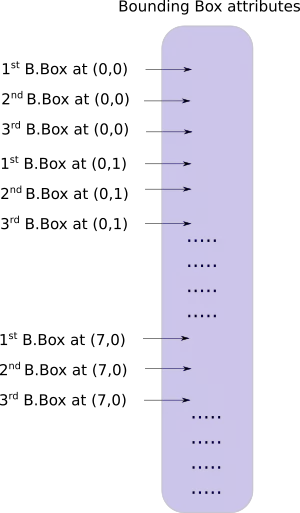`` batch_size = prediction.size(0) stride = inp_dim // prediction.size(2) grid_size = inp_dim // stride bbox_attrs = 5 + num_classes num_anchors = len(anchors) prediction = prediction.view(batch_size, bbox_attrs*num_anchors, grid_size*grid_size) prediction = prediction.transpose(1,2).contiguous() prediction = prediction.view(batch_size, grid_size*grid_size*num_anchors, bbox_attrs)``

`` anchors = [(a/stride, a/stride) for a in anchors]``

`` #Sigmoid the centre_X, centre_Y. and object confidencce prediction[:,:,0] = torch.sigmoid(prediction[:,:,0]) prediction[:,:,1] = torch.sigmoid(prediction[:,:,1]) prediction[:,:,4] = torch.sigmoid(prediction[:,:,4])``

`` #Add the center offsets grid = np.arange(grid_size) a,b = np.meshgrid(grid, grid) x_offset = torch.FloatTensor(a).view(-1,1) y_offset = torch.FloatTensor(b).view(-1,1) if CUDA: x_offset = x_offset.cuda() y_offset = y_offset.cuda() x_y_offset = torch.cat((x_offset, y_offset), 1).repeat(1,num_anchors).view(-1,2).unsqueeze(0) prediction[:,:,:2] += x_y_offset``

`` #log space transform height and the width anchors = torch.FloatTensor(anchors) if CUDA: anchors = anchors.cuda() anchors = anchors.repeat(grid_size*grid_size, 1).unsqueeze(0) prediction[:,:,2:4] = torch.exp(prediction[:,:,2:4])*anchors``

`` prediction[:,:,5: 5 + num_classes] = torch.sigmoid((prediction[:,:, 5 : 5 + num_classes]))``

``prediction[:,:,:4] *= stride``

loop 部分到這裏就大致結束了。

`` return prediction``

``from util import * ``

`` elif module_type == 'yolo':  anchors = self.module_list[i].anchors #Get the input dimensions inp_dim = int (self.net_info["height"]) #Get the number of classes num_classes = int (module["classes"]) #Transform  x = x.data x = predict_transform(x, inp_dim, anchors, num_classes, CUDA) if not write: #if no collector has been intialised.  detections = x write = 1 else:  detections = torch.cat((detections, x), 1) outputs[i] = x``

`` return detections``

``wget https://github.com/ayooshkathuria/pytorch-yolo-v3/raw/master/dog-cycle-car.png``

``def get_test_input(): img = cv2.imread("dog-cycle-car.png") img = cv2.resize(img, (416,416)) #Resize to the input dimension img_ = img[:,:,::-1].transpose((2,0,1)) # BGR -> RGB | H X W C -> C X H X W  img_ = img_[np.newaxis,:,:,:]/255.0 #Add a channel at 0 (for batch) | Normalise img_ = torch.from_numpy(img_).float() #Convert to float img_ = Variable(img_) # Convert to Variable return img_``

``model = Darknet("cfg/yolov3.cfg")inp = get_test_input()pred = model(inp)print (pred)``

``( 0 ,.,.) =  16.0962 17.0541 91.5104 ... 0.4336 0.4692 0.5279 15.1363 15.2568 166.0840 ... 0.5561 0.5414 0.5318 14.4763 18.5405 409.4371 ... 0.5908 0.5353 0.4979 ⋱ ...  411.2625 412.0660 9.0127 ... 0.5054 0.4662 0.5043 412.1762 412.4936 16.0449 ... 0.4815 0.4979 0.4582 412.1629 411.4338 34.9027 ... 0.4306 0.5462 0.4138[torch.FloatTensor of size 1x10647x85]``

``wget https://pjreddie.com/media/files/yolov3.weights``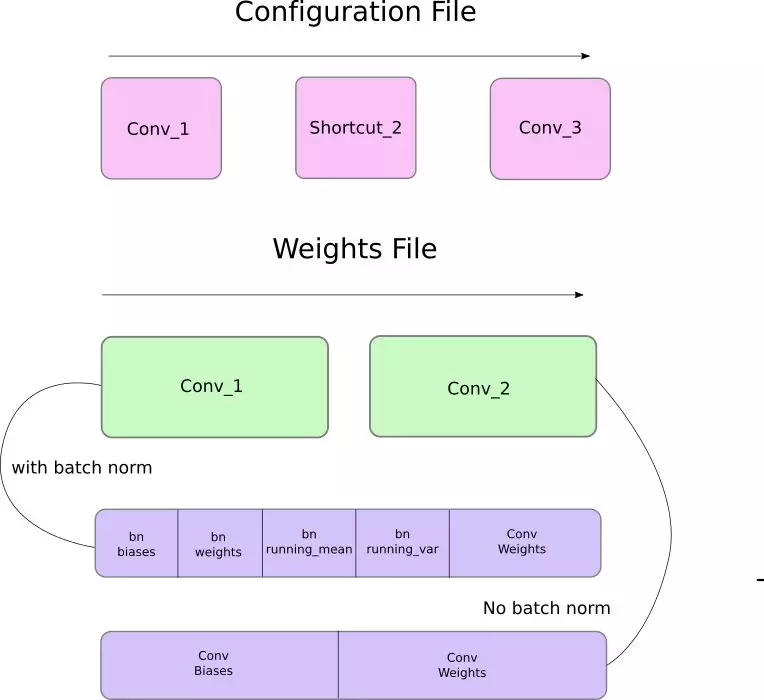``def load_weights(self, weightfile):``

`` #Open the weights file fp = open(weightfile, "rb") #The first 5 values are header information  # 1. Major version number # 2. Minor Version Number # 3. Subversion number  # 4,5. Images seen by the network (during training) header = np.fromfile(fp, dtype = np.int32, count = 5) self.header = torch.from_numpy(header) self.seen = self.header``

`` weights = np.fromfile(fp, dtype = np.float32)``

`` ptr = 0 for i in range(len(self.module_list)): module_type = self.blocks[i + 1]["type"] #If module_type is convolutional load weights #Otherwise ignore.``

`` if module_type == "convolutional": model = self.module_list[i] try: batch_normalize = int(self.blocks[i+1]["batch_normalize"]) except: batch_normalize = 0 conv = model``

`` if (batch_normalize): bn = model #Get the number of weights of Batch Norm Layer num_bn_biases = bn.bias.numel() #Load the weights bn_biases = torch.from_numpy(weights[ptr:ptr + num_bn_biases]) ptr += num_bn_biases bn_weights = torch.from_numpy(weights[ptr: ptr + num_bn_biases]) ptr += num_bn_biases bn_running_mean = torch.from_numpy(weights[ptr: ptr + num_bn_biases]) ptr += num_bn_biases bn_running_var = torch.from_numpy(weights[ptr: ptr + num_bn_biases]) ptr += num_bn_biases #Cast the loaded weights into dims of model weights.  bn_biases = bn_biases.view_as(bn.bias.data) bn_weights = bn_weights.view_as(bn.weight.data) bn_running_mean = bn_running_mean.view_as(bn.running_mean) bn_running_var = bn_running_var.view_as(bn.running_var) #Copy the data to model bn.bias.data.copy_(bn_biases) bn.weight.data.copy_(bn_weights) bn.running_mean.copy_(bn_running_mean) bn.running_var.copy_(bn_running_var)``

`` else: #Number of biases num_biases = conv.bias.numel() #Load the weights conv_biases = torch.from_numpy(weights[ptr: ptr + num_biases]) ptr = ptr + num_biases #reshape the loaded weights according to the dims of the model weights conv_biases = conv_biases.view_as(conv.bias.data) #Finally copy the data conv.bias.data.copy_(conv_biases)``

``#Let us load the weights for the Convolutional layersnum_weights = conv.weight.numel()#Do the same as above for weightsconv_weights = torch.from_numpy(weights[ptr:ptr+num_weights])ptr = ptr + num_weightsconv_weights = conv_weights.view_as(conv.weight.data)conv.weight.data.copy_(conv_weights)``

``model = Darknet("cfg/yolov3.cfg")model.load_weights("yolov3.weights")``